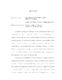## Quantum Detection and Finite Frames

 dc.contributor.advisor Benedetto, John J en_US dc.contributor.author Kebo, Andrew Kei en_US dc.contributor.department Mathematics en_US dc.contributor.publisher Digital Repository at the University of Maryland en_US dc.contributor.publisher University of Maryland (College Park, Md.) en_US dc.date.accessioned 2005-08-03T13:47:36Z dc.date.available 2005-08-03T13:47:36Z dc.date.issued 2005-04-12 en_US dc.description.abstract In quantum mechanics, the definition of a Von Neumann measurement can be generalized using positive-operator-valued measures. This modified definition of a quantum measurement allows one to better distinguish between a set of nonorthogonal quantum states. In this thesis we examine a quantum detection problem, where we have a physical system whose state is limited to be in only one of a finite number of possibilities. These possible states are not necessarily orthogonal. We want to find the best method of measuring the system in order to distinguish which state the system is in. Mathematically, we want to find a positive-operator-valued measure that minimizes the probability of a detection error. It is shown that all tight-frames with frame constant 1 correspond to positive-operator-valued measures. We reformulate the problem in terms of tight-frames that minimize the detection error. In the finite dimensional case, the problem of finding the tight-frame that minimizes the error can be converted into a Hamiltonian system on the group $SO(N)$. The minimum energy solutions of this Hamiltonian system correspond exactly to the tight-frames that minimize the detection error. In this setting, several numerical methods can be applied to give numerical constructions of the desired tight-frames. en_US dc.format.extent 490794 bytes dc.format.mimetype application/pdf dc.identifier.uri http://hdl.handle.net/1903/2385 dc.language.iso en_US dc.subject.pqcontrolled Mathematics en_US dc.subject.pquncontrolled finite frames en_US dc.subject.pquncontrolled quantum detection en_US dc.subject.pquncontrolled positive-operator-valued measures en_US dc.title Quantum Detection and Finite Frames en_US dc.type Dissertation en_US
##### Original bundle
Now showing 1 - 1 of 1